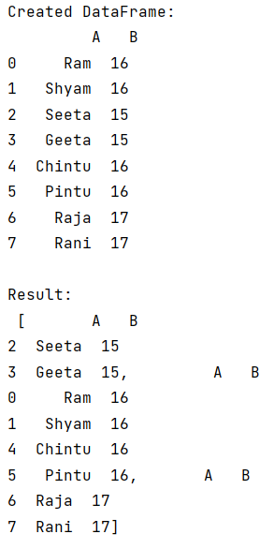# Python - Split pandas dataframe based on groupby

Given a Pandas DataFrame, we have to split pandas dataframe based on groupby.
Submitted by Pranit Sharma, on July 28, 2022

Pandas is a special tool that allows us to perform complex manipulations of data effectively and efficiently. Inside pandas, we mostly deal with a dataset in the form of DataFrame. DataFrames are 2-dimensional data structures in pandas. DataFrames consist of rows, columns, and data.

Sometimes, while dealing with a large DataFrame, we have some common values and their corresponding column values are unique, in this case, you want to split the data frame based on the common values which are grouped.

For example, you are having two columns A and B, A has all the unique values but B has some common values, after grouping all the common values of B, we want to split the data frame, with one new column for each unique value of B. For this purpose, we will first use pandas.DataFrame.groupby() method.

The pandas.DataFrame.groupby() is a simple but very useful concept in pandas. By using groupby, we can create a grouping of certain values and perform some operations on those values. The pandas.DataFrame.groupby() method split the object, apply some operations, and then combines them to create a group hence a large amount of data and computations can be performed on these groups.

Let us understand with the help of an example,

## Python code to split pandas dataframe based on groupby

```# Importing Pandas package
import pandas as pd

# Creating dictionary
d = {
'A':['Ram','Shyam','Seeta','Geeta','Chintu','Pintu','Raja','Rani'],
'B':[16,16,15,15,16,16,17,17]
}

# Creating DataFrame
df = pd.DataFrame(d)

# Display original DataFrame
print("Created DataFrame:\n",df,"\n")

# Using groupby
Result = df.groupby('B')

# Splitting the dataframe
Splitted_DataFrame = [Result.get_group(x) for x in Result.groups]

# Display
print("Result:\n",Splitted_DataFrame)
```

Output: